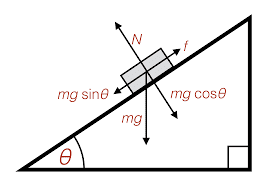# Forces acting on a car on an incline

TheBigDig
Homework Statement:
A 1100 kg car is parked on an incline of 1 in 30. What is the normal force acting on the car? In the absence of friction what would be the acceleration if the handbrake were released?
Relevant Equations:
## \vec{F} = \mu_k \vec{N} ##
## F = mgsin\alpha ##
## N = mgcos\alpha ##
For part 1, I got ## tan \alpha = 1/30 ##
##\alpha = 1.9^{\circ}##
##mgcos(1.9) = 10774N##

I'm a little thrown off by the second part. Are we supposed to assume that in the absence of friction, F = N and then substitute F = ma to solve for this?

Homework Helper
Gold Member
2022 Award
Homework Statement:: A 1100 kg car is parked on an incline of 1 in 30. What is the normal force acting on the car? In the absence of friction what would be the acceleration if the handbrake were released?
Relevant Equations:: ## \vec{F} = \mu_k \vec{N} ##
## F = mgsin\alpha ##
## F = mgcos\alpha ##

F = N
It would help if you were to distinguish forces in different directions instead of writing "F" in every case.
Which direction is the normal force? In which direction will the car move if the brake is released?

TheBigDig
It would help if you were to distinguish forces in different directions instead of writing "F" in every case.
Which direction is the normal force? In which direction will the car move if the brake is released?
Sorry yes of course. The normal force is perpendicular to the surface and the car will roll backward. I believe the model should correspond to thisJames2020
The force acting parallel to the slope causes the car’s acceleration so, since F=ma, you have mgsin(θ)=ma so a=gsin(θ).

•